# 1,65 % p.a. is the maximal interest rate under the term deposits for 3 months02.07.2020

## Bankchart.com.au has surveyed the terms of term deposits for 3 months in the national currency. The survey covered all the deposits with any form of interests repayment, regardless of the replenishment option or the deposit partial withdrawal option. Our website's analysts have studied the loan offers from all Australian banks. Review date - 02.07.2020

As of 23 June 2020 the deposits under review have been offered by 32 banks.  The average interest rate under such deposits equals 0,84 % p.a. The total number of the deposits programs available for the specified terms is 74.

The maximal return among the deposits under review is offered by Arab Bank. The interest rate under "Fixed Term Deposit" deposit program constitutes 1,65 % p.a. The table below shows the best offers by each bank selected by Bankchart.com.au (in terms of profitability) for the aforementioned terms.

### The TOP-32 term deposits for 3 months, as of 23 June 2020

#

Name

Interest Rate, %

1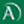Arab Bank

«Fixed Term Deposit»

1,65

interest rate, %

rate type

fixed

interest periodicity

at maturity

minimum sum

\$ 50 000,00

2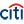Citibank

«Term deposit»

1,35

interest rate, %

rate type

fixed

interest periodicity

monthly

minimum sum

\$ 75 000,00

3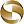Mega International Commercial Bank

«Term Deposits»

1,30

interest rate, %

rate type

fixed

interest periodicity

at maturity

minimum sum

\$ 1 000,00

4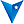BankVic

«Term deposit»

1,25

interest rate, %

rate type

fixed

interest periodicity

at maturity

minimum sum

\$ 5 000,00

5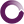Beyond Bank Australia

«Term Deposits»

1,25

interest rate, %

rate type

fixed

interest periodicity

at maturity

minimum sum

\$ 200 000,00

6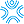Bank First

«Term Deposits»

1,25

interest rate, %

rate type

fixed

interest periodicity

monthly

minimum sum

\$ 50 000,00

7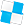Police Bank

«Term Deposits»

1,20

interest rate, %

rate type

fixed

interest periodicity

at maturity

minimum sum

\$ 10 000,00

8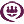RACQ

«Term Deposit»

1,20

interest rate, %

rate type

fixed

interest periodicity

monthly

minimum sum

\$ 1 000,00

9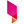Bank Australia

«Term Deposits»

1,10

interest rate, %

rate type

fixed

interest periodicity

at maturity

minimum sum

\$ 500,00

10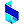Bank of Sydney

«Online Term Deposit»

1,10

interest rate, %

rate type

fixed

interest periodicity

at maturity

minimum sum

\$ 50 000,00

11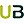Ubank

«Term deposit»

1,10

interest rate, %

rate type

variable

interest periodicity

at maturity

minimum sum

\$ 0,00

12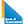Bank of Queensland

«Term deposit»

1,00

interest rate, %

rate type

fixed

interest periodicity

at maturity

minimum sum

\$ 5 000,00

13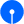State Bank of India

«Term Deposits»

0,95

interest rate, %

rate type

fixed

interest periodicity

at maturity

minimum sum

\$ 100,00

14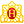Heritage Bank

«Term Deposits»

0,95

interest rate, %

rate type

fixed

interest periodicity

at maturity

minimum sum

\$ 1 000,00

15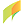G&C Mutual Bank

«Term Deposits»

0,95

interest rate, %

rate type

fixed

interest periodicity

at maturity, annually

minimum sum

\$ 1 000,00

16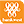Bank of Western Australia

«Online Term Deposit»

0,95

interest rate, %

rate type

fixed

interest periodicity

at maturity, annually

minimum sum

\$ 5 000,00

17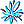AMP Bank

«Term Deposits»

0,95

interest rate, %

rate type

fixed

interest periodicity

at maturity

minimum sum

\$ 25 000,01

18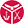Defence Bank

«Term Deposits»

0,90

interest rate, %

rate type

fixed

interest periodicity

at maturity

minimum sum

\$ 1 000,00

19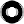Macquarie Bank

«Term Deposit»

0,90

interest rate, %

rate type

fixed

interest periodicity

monthly

minimum sum

\$ 0,00

20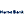Hume Bank

«Term Deposits»

0,90

interest rate, %

rate type

fixed

interest periodicity

at maturity

minimum sum

\$ 5 000,00

21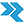Rural Bank

«Short Term Deposit»

0,80

interest rate, %

rate type

fixed

interest periodicity

monthly

minimum sum

\$ 5 000,00

22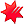National Australia Bank

«NAB Term Deposits»

0,80

interest rate, %

rate type

fixed

interest periodicity

at maturity

minimum sum

\$ 5 000,00

23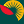Suncorp Bank

«Term Deposit»

0,80

interest rate, %

rate type

fixed

interest periodicity

at maturity

minimum sum

\$ 1 000 000,00

24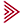Bendigo and Adelaide Bank

«Term deposit»

0,75

interest rate, %

rate type

fixed

interest periodicity

at maturity

minimum sum

\$ 5 000,00

25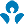Australia and New Zealand (ANZ)

0,75

interest rate, %

rate type

fixed

interest periodicity

at maturity

minimum sum

\$ 5 000,00

26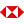HSBC Bank

«Term Deposits»

0,70

interest rate, %

rate type

fixed

interest periodicity

at maturity

minimum sum

\$ 5 000,00

27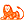ING Direct

«Personal Term Deposit»

0,70

interest rate, %

rate type

fixed

interest periodicity

at maturity

minimum sum

\$ 10 000,00

28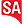Bank SA

«Term Deposits»

0,70

interest rate, %

rate type

fixed

interest periodicity

at maturity

minimum sum

\$ 1 000,00

29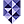Bank of Melbourne

«Term Deposits»

0,70

interest rate, %

rate type

fixed

interest periodicity

at maturity

minimum sum

\$ 1 000,00

30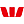Westpac Bank

«Term Deposit»

0,70

interest rate, %

rate type

fixed

interest periodicity

monthly

minimum sum

\$ 5 000,00

31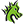St. George Bank

«Term Deposits»

0,70

interest rate, %

rate type

fixed

interest periodicity

at maturity

minimum sum

\$ 1 000,00

32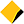Commonwealth Bank of Australia

«Term Deposits»

0,65

interest rate, %

rate type

fixed

interest periodicity

annually, every 6 months

minimum sum

\$ 49 999,00

To view the up-to-date rating of the deposits at our website here.